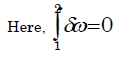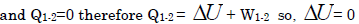Courses

# Basic Concepts Of Thermodynamics - MCQ Test 1

## 20 Questions MCQ Test Mock Test Series - Mechanical Engineering (ME) for GATE 2020 | Basic Concepts Of Thermodynamics - MCQ Test 1

Description
This mock test of Basic Concepts Of Thermodynamics - MCQ Test 1 for Mechanical Engineering helps you for every Mechanical Engineering entrance exam. This contains 20 Multiple Choice Questions for Mechanical Engineering Basic Concepts Of Thermodynamics - MCQ Test 1 (mcq) to study with solutions a complete question bank. The solved questions answers in this Basic Concepts Of Thermodynamics - MCQ Test 1 quiz give you a good mix of easy questions and tough questions. Mechanical Engineering students definitely take this Basic Concepts Of Thermodynamics - MCQ Test 1 exercise for a better result in the exam. You can find other Basic Concepts Of Thermodynamics - MCQ Test 1 extra questions, long questions & short questions for Mechanical Engineering on EduRev as well by searching above.
QUESTION: 1

Solution:
QUESTION: 2

Solution:
QUESTION: 3

### A balloon containing an ideal gas is initially kept in an evacuated and insulated room. The balloon ruptures and the gas fills up the entire room. Which one of the following statements is TRUE at the end of above process?

Solution:

It is free expansion. Since vacuum does not offer any resistance, there is no work transfer involved in free expansion.QUESTION: 4

A system comprising a single phase is called a

Solution:
QUESTION: 5

The main cause of the irreversibility is

Solution:
QUESTION: 6

Which of the following are intensive properties?

1. Kinetic Energy

2. Specific Enthalpy

3. Pressure

4. Entropy

Select the correct answer using the code given below:

Solution:

Option (b) 2 and 3 is  correct.

Specific enthalpy and pressure

Are both intensive properties.

Explanation:-

{ Intensive properties are those that do not depend on the size of the system. Specific enthalpy is the enthalpy on a per gram basis. Since  this value is normalized to the per gram basis it does not depend on the size of the system being measured. }
So, both are intensive properties.

QUESTION: 7

Consider the following:

1. Kinetic energy

2. Entropy

3. Thermal conductivity

4. Pressure

Which of these are intensive properties?

Solution:

Kinetic energy 1/2mv2 depends on mass, Entropy kJ/k depends on mass so Entropy is extensive property but specific entropy kJ/kg K is an intensive property.

QUESTION: 8

Consider the following properties:

1. Entropy

2. Viscosity

3. Temperature

4. Specific heat at constant volume

Which of the above properties of a system is/are extensive?

Solution:

Extensive property: Whose value depends on the size or extent i.e. mass of the system (upper case letters as the symbols) e.g., Volume, Mass (V, M). If mass is increased, the value of extensive property also increases.

QUESTION: 9

In highly rarefied gases, the concept of this loses validity

Solution:
QUESTION: 10

A control volume is

Solution:

A control volume is a mathematical abstraction employed in the process of creating mathematical models of physical processes. In an inertial frame of reference, it is a volume fixed in space or moving with constant flow velocity through which the continuum (gas, liquid or solid) flows. The surface enclosing the control volume is referred to as the control surface.

At steady state, a control volume can be thought of as an arbitrary volume in which the mass of the continuum remains constant. As a continuum moves through the control volume, the mass entering the control volume is equal to the mass leaving the control volume. At steady state, and in the absence of work and heat transfer, the energy within the control volume remains constant. It is analogous to the classical mechanics concept of the free body diagram.

QUESTION: 11

Isothermal compression of air in a Stirling engine is an example of

Solution:
QUESTION: 12

Assertion (A): In thermodynamic analysis, the concept of reversibility is that, a reversible process is the most efficient process.

Reason (R): The energy transfer as heat and work during the forward process as always identically equal to the energy transfer is heat and work during the reversal or the process.

Solution:

The energy transfer as heat and work during the forward process as always
identically equal to the energy transfer is heat and work during the reversal or the process is the correct reason for maximum efficiency because it is conservative system.

QUESTION: 13

Ice kept in a well insulated thermo flask is an example of which system?

Solution:

Isolated System - in which there is no interaction between system and the
surroundings. It is of fixed mass and energy, and hence there is no mass and energy transfer across the system boundary.

QUESTION: 14

A thermodynamic system is considered to be an isolated one if

Solution:
QUESTION: 15

Measurement of temperature is based on which law of thermodynamics?

Solution:

All temperature measurements are based on Zeroth law of thermodynamics.

QUESTION: 16

Zeroth Law of thermodynamics states that

Solution:

The Zeroth law of thermodynamics is the basis for measurement of temperature and setting its scale. In simple word, Zeroth law of thermodynamics says that “When two bodies are separately in thermal equilibrium with the third body, then the two are also in thermal equilibrium with each other."

QUESTION: 17

The constant volume gas thermometer works on the principle that

Solution:

We know that PV=mRT and it holds good at low pressure and high temperature. If volume is constant P is proportional to T (since R is constant and m depends on gas and is also constant). Therefore, at low pressure, the temperature of gas is proportional to its pressure at constant volume.

QUESTION: 18

Assertion (a): If an alcohol and a mercury thermometer read exactly 0°C at the ice point and 100°C at the steam point and the distance between the two points is divided into 100 equal parts in both thermometers, the two thermometers will give exactly the same reading at 50°C.

Reason (R): Temperature scales are arbitrary.

Solution:

Because firstly they are of different material they have different thermal expansion etc and secondly no 2 things whether of same company can tel u the same.
Eg. if u purchase the weighing machine of 2 companies even they can' t tell the same weight .. i.e some amt of deflection will be there

QUESTION: 19

Pressure reaches a value of absolute zero

Solution:

Absolute zero, temperature at which a thermodynamic system has the lowest energy. It appeared that an “ideal gas” at constant pressure would reach zero volume at what is now called the absolute zero of temperature.

QUESTION: 20

Assertion (A): Thermodynamic work is path-dependent except for an adiabatic process.

Reason(R): It is always possible to take a system from a given initial state to any final state by performing adiabatic work only.

Solution: